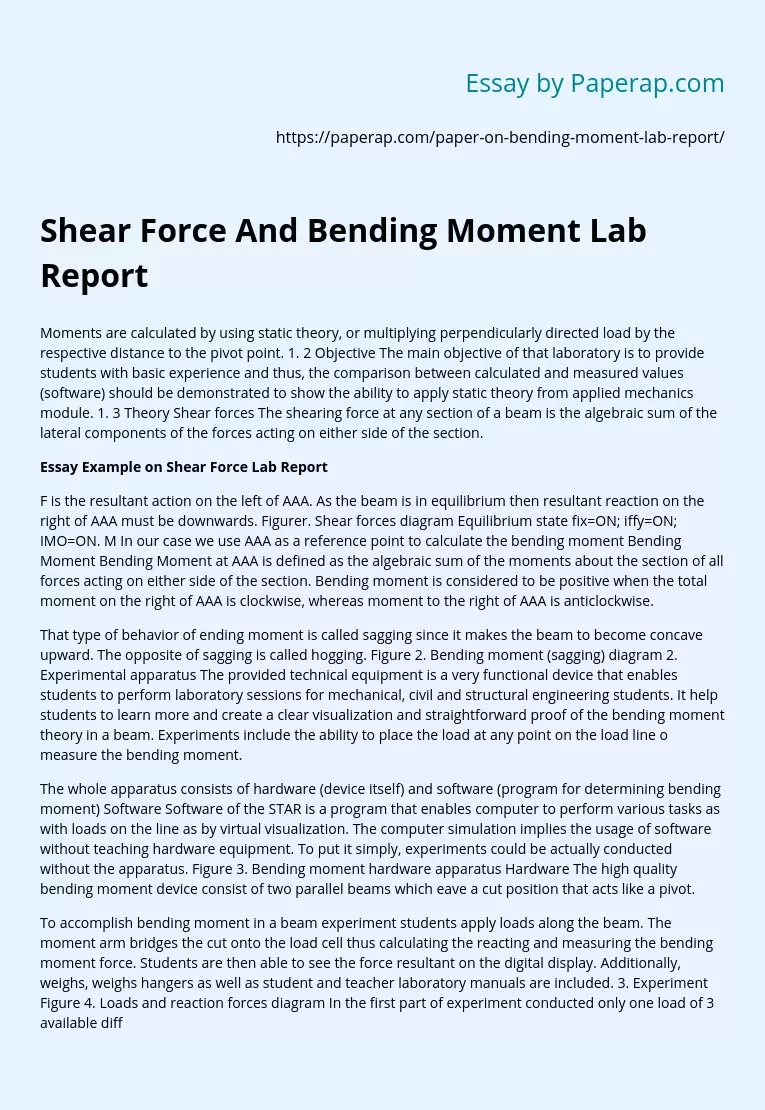# Shear Force And Bending Moment Lab Report

Moments are calculated by using static theory, or multiplying perpendicularly directed load by the respective distance to the pivot point. 1. 2 Objective The main objective of that laboratory is to provide students with basic experience and thus, the comparison between calculated and measured values (software) should be demonstrated to show the ability to apply static theory from applied mechanics module. 1. 3 Theory Shear forces The shearing force at any section of a beam is the algebraic sum of the lateral components of the forces acting on either side of the section.

## Essay Example on Shear Force Lab Report

F is the resultant action on the left of AAA. As the beam is in equilibrium then resultant reaction on the right of AAA must be downwards. Figurer. Shear forces diagram Equilibrium state fix=ON; iffy=ON; IMO=ON. M In our case we use AAA as a reference point to calculate the bending moment Bending Moment Bending Moment at AAA is defined as the algebraic sum of the moments about the section of all forces acting on either side of the section. Bending moment is considered to be positive when the total moment on the right of AAA is clockwise, whereas moment to the right of AAA is anticlockwise.

That type of behavior of ending moment is called sagging since it makes the beam to become concave upward. The opposite of sagging is called hogging. Figure 2. Bending moment (sagging) diagram 2. Experimental apparatus The provided technical equipment is a very functional device that enables students to perform laboratory sessions for mechanical, civil and structural engineering students.

Get quality help nowSweet VVerified

Proficient in: Force4.9 (984)

“ Ok, let me say I’m extremely satisfy with the result while it was a last minute thing. I really enjoy the effort put in. ”+84 relevant experts are online

It help students to learn more and create a clear visualization and straightforward proof of the bending moment theory in a beam. Experiments include the ability to place the load at any point on the load line o measure the bending moment.

The whole apparatus consists of hardware (device itself) and software (program for determining bending moment) Software Software of the STAR is a program that enables computer to perform various tasks as with loads on the line as by virtual visualization. The computer simulation implies the usage of software without teaching hardware equipment. To put it simply, experiments could be actually conducted without the apparatus. Figure 3. Bending moment hardware apparatus Hardware The high quality bending moment device consist of two parallel beams which eave a cut position that acts like a pivot.

To accomplish bending moment in a beam experiment students apply loads along the beam. The moment arm bridges the cut onto the load cell thus calculating the reacting and measuring the bending moment force. Students are then able to see the force resultant on the digital display. Additionally, weighs, weighs hangers as well as student and teacher laboratory manuals are included. 3. Experiment Figure 4. Loads and reaction forces diagram In the first part of experiment conducted only one load of 3 available different dads were applied at the distance of mm from the left corner of beam (0-PI).